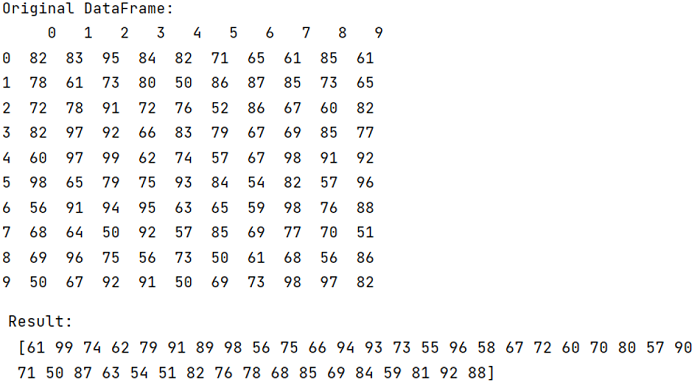# Find unique values in a pandas dataframe, irrespective of row or column location

Given a pandas dataframe, we have to find the unique values, irrespective of row or column location.
Submitted by Pranit Sharma, on September 17, 2022

Pandas is a special tool that allows us to perform complex manipulations of data effectively and efficiently. Inside pandas, we mostly deal with a dataset in the form of DataFrame. DataFrames are 2-dimensional data structures in pandas. DataFrames consist of rows, columns, and data.

## Finding unique values, irrespective of row or column location

Suppose we have a pandas DataFrame, by this, we mean that if there are 50 unique values in a DataFrame then we need to find those unique values and not the total count of unique values.

For this purpose, we will first use df.values.ravel() method upon which we will use unique() method. The ravel() function returns the flattened underlying data as a ndarray.

Let us understand with the help of an example,

## Python code to find the unique values in a pandas dataframe, irrespective of row or column location

```# Importing pandas package
import pandas as pd

# Importing numpy package
import numpy as np

# Creating a DataFrame
df = pd.DataFrame(np.random.randint(50,100,size=100).reshape(10,10))

# Display original Datarame
print("Original DataFrame:\n",df,"\n")

# Getting unique values
res =  pd.Series(df.values.ravel()).unique()

# Display result
print("Result:\n",res)
```

Output:What's New (MCQs)

Top Interview Coding Problems/Challenges!

Languages: » C » C++ » C++ STL » Java » Data Structure » C#.Net » Android » Kotlin » SQL
Web Technologies: » PHP » Python » JavaScript » CSS » Ajax » Node.js » Web programming/HTML
Solved programs: » C » C++ » DS » Java » C#
Aptitude que. & ans.: » C » C++ » Java » DBMS
Interview que. & ans.: » C » Embedded C » Java » SEO » HR
CS Subjects: » CS Basics » O.S. » Networks » DBMS » Embedded Systems » Cloud Computing
» Machine learning » CS Organizations » Linux » DOS
More: » Articles » Puzzles » News/Updates

© https://www.includehelp.com some rights reserved.